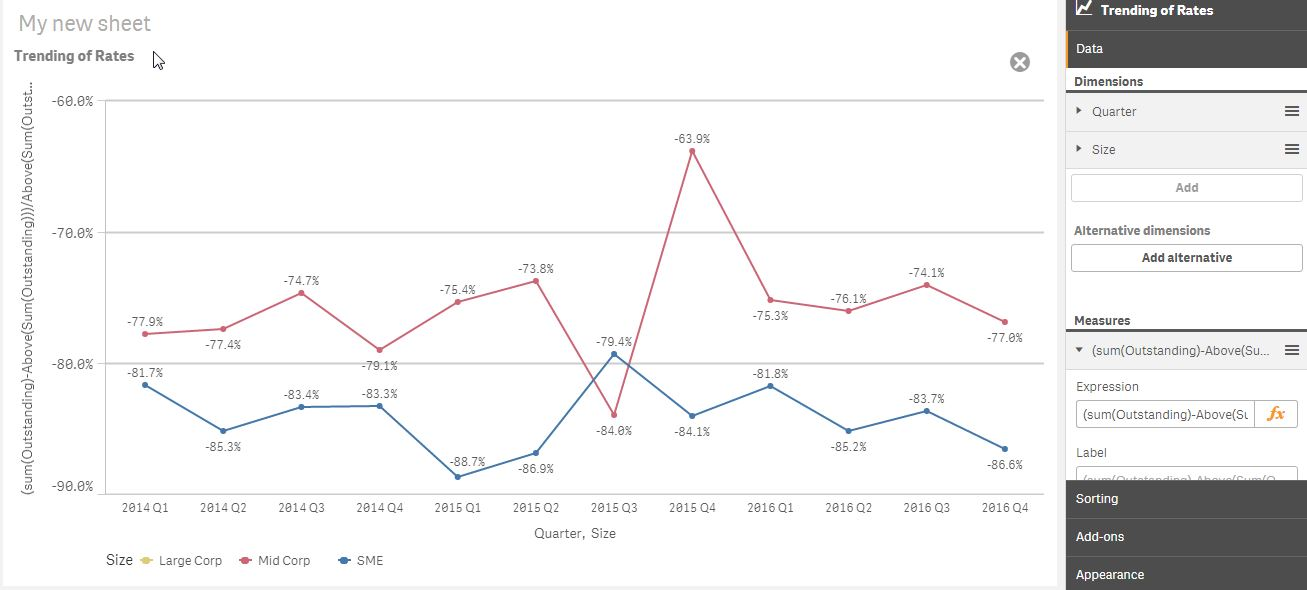# Qlik Sense App Development

Announcements
BI & Data Trends 2021. Discover the top 10 trends emerging in today. Join us on Dec. 8th REGISTER
cancel
Showing results for
Did you mean:
HighlightedNot applicable

## Problem facing when using Above(), Multi DimensionAs you can see from the picture, the line for "Large Corp" disappeared because of the usage of Above function.

I want to specific the calculation using above function refer to dimension "Quarter" and then split by Dimension "Size".

Is there any solution for this?

This is the formula of measure : (sum(Outstanding)-Above(Sum(Outstanding)))/Above(Sum(Outstanding))

1 Solution

Accepted Solutions
HighlightedMVP

Try this:

Aggr((Sum(Outstanding) - Above(Sum(Outstanding)))/Above(Sum(Outstanding)), Size, Quarter)

3 Replies
HighlightedMVP

Try this:

Aggr((Sum(Outstanding) - Above(Sum(Outstanding)))/Above(Sum(Outstanding)), Size, Quarter)

HighlightedMVP

Or this:

Aggr((Sum(Outstanding) - Above(Sum(Outstanding)))/Above(Sum(Outstanding)), Size, (Quarter, (TEXT, ASCENDING)))

HighlightedNot applicable

Thanks a lot. I gt it right.